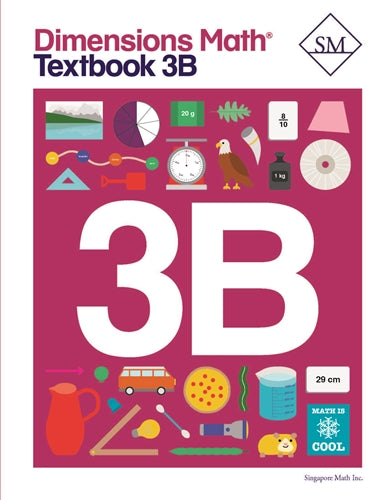# Dimensions Math Textbook 3B

Dimensions Math® Textbook 3B

Chapter 8: Multiplying and Dividing with 6, 7, 8, and 9

Lesson 1: The Multiplication Table of 6
Lesson 2: The Multiplication Table of 7
Lesson 3: Multiplying by 6 and 7
Lesson 4: Dividing by 6 and 7
Lesson 5: Practice A
Lesson 6: The Multiplication Table of 8
Lesson 7: The Multiplication Table of 9
Lesson 8: Multiplying by 8 and 9
Lesson 9: Dividing by 8 and 9
Lesson 10: Practice B

Chapter 9: Fractions — Part 1

Lesson 1: Fractions of a Whole
Lesson 2: Fractions on a Number Line
Lesson 3: Comparing Fractions with Like Denominators
Lesson 4: Comparing Fractions with Like Numerators

Lesson 5: Practice

Chapter 10: Fractions — Part 2

Lesson 1: Equivalent Fractions
Lesson 2: Finding Equivalent Fractions
Lesson 3: Simplifying Fractions
Lesson 4: Comparing Fractions — Part 1
Lesson 5: Comparing Fractions — Part 2
Lesson 6: Practice A
Lesson 7: Adding and Subtracting Fractions — Part 1
Lesson 8: Adding and Subtracting Fractions — Part 2
Lesson 9: Practice B

Chapter 11: Measurement

Lesson 1: Meters and Centimeters
Lesson 2: Subtracting from Meters
Lesson 3: Kilometers
Lesson 4: Subtracting from Kilometers
Lesson 5: Liters and Milliliters
Lesson 6: Kilograms and Grams
Lesson 7: Word Problems
Lesson 8: Practice
Review 3

Chapter 12: Geometry

Lesson 1: Circles
Lesson 2: Angles
Lesson 3: Right Angles
Lesson 4: Triangles
Lesson 5: Properties of Triangles
Lesson 7: Using a Compass
Lesson 8: Practice

Chapter 13: Area and Perimeter

Lesson 1: Area
Lesson 2: Units of Area
Lesson 3: Area of Rectangles
Lesson 4: Area of Composite Figures
Lesson 5: Practice A
Lesson 6: Perimeter
Lesson 7: Perimeter of Rectangles
Lesson 8: Area and Perimeter
Lesson 9: Practice B

Chapter 14: Time

Lesson 1: Units of Time
Lesson 2: Calculating Time — Part 1
Lesson 3: Practice A
Lesson 4: Calculating Time — Part 2
Lesson 5: Calculating Time — Part 3
Lesson 6: Calculating Time — Part 4
Lesson 7: Practice B

Chapter 15: Money

Lesson 1: Dollars and Cents
Lesson 2: Making \$10
Lesson 4: Subtracting Money
Lesson 5: Word Problems
Lesson 6: Practice

Review 4
Review 5

Dimensions Math® PreK-5 series features the progression, rigor, and pacing that define Singapore math. Throughout the series, five characters offer students suggestions on how to think about problems. They remind students of strategies they’ve learned and point out important information that encourages them to come up with their own solutions.

Textbook lessons begin with a task that allows students to apply their previous knowledge and learn through discussion. Once students have mastered a concept with the use of concrete and pictorial aids, they are ready to take on more abstract mathematical problem sets. They reach fluency by collecting various strategies along the way and applying them to new problems. Word problems give students a sense of math in real-world contexts.

Workbooks offer independent practice that follows a careful progression of exercise variation. Each textbook lesson includes a corresponding workbook exercise that starts with pictorial representation and progresses to more challenging abstract problems. Workbooks for PreK-2 are perforated.

Teacher’s Guides include lesson plans, mathematical background, games, helpful suggestions, and comprehensive resources for daily lessons. Lessons are laid out clearly and activities are designed for the whole class, small groups, and extension.

Textbooks and Workbooks do not include answer keys. Answers are in Teacher's Guides.
Textbook:
ISBN  9781947226098